• pr曲线
2022-04-22 06:10:28

# mmdetection 绘制PR曲线

参考：https://github.com/xuhuasheng/mmdetection_plot_pr_curve

import os
import mmcv
import numpy as np
import matplotlib.pyplot as plt

from pycocotools.coco import COCO
from pycocotools.cocoeval import COCOeval

from mmcv import Config
from mmdet.datasets import build_dataset

def getPRArray(config_file, result_file, metric="bbox"):
"""plot precison-recall curve based on testing results of pkl file.

Args:
config_file (list[list | tuple]): config file path.
result_file (str): pkl file of testing results path.
metric (str): Metrics to be evaluated. Options are
'bbox', 'segm'.
"""

cfg = Config.fromfile(config_file)
# turn on test mode of dataset
if isinstance(cfg.data.test, dict):
cfg.data.test.test_mode = True
elif isinstance(cfg.data.test, list):
for ds_cfg in cfg.data.test:
ds_cfg.test_mode = True

# build dataset
dataset = build_dataset(cfg.data.test)
# load result file in pkl format
# convert pkl file (list[list | tuple | ndarray]) to json
json_results, _ = dataset.format_results(pkl_results)
# initialize COCO instance
coco = COCO(annotation_file=cfg.data.test.ann_file)
coco_gt = coco
# initialize COCOeval instance
coco_eval = COCOeval(coco_gt, coco_dt, metric)
coco_eval.evaluate()
coco_eval.accumulate()
coco_eval.summarize()
# extract eval data
precisions = coco_eval.eval["precision"]
'''
precisions[T, R, K, A, M]
T: iou thresholds [0.5 : 0.05 : 0.95], idx from 0 to 9
R: recall thresholds [0 : 0.01 : 1], idx from 0 to 100
K: category, idx from 0 to ...
A: area range, (all, small, medium, large), idx from 0 to 3
M: max dets, (1, 10, 100), idx from 0 to 2
'''

return precisions

'''
out为输出的图片名字
'''
def PR(config, result, out):
precisions = getPRArray(config, result)

pr_array1 = precisions[0, :, 0, 0, 2]   # IOU = 0.5
pr_array2 = precisions[1, :, 0, 0, 2]   # IOU = 0.55
pr_array3 = precisions[2, :, 0, 0, 2]   # IOU = 0.6
pr_array4 = precisions[3, :, 0, 0, 2]   # IOU = 0.65
pr_array5 = precisions[4, :, 0, 0, 2]   # IOU = 0.7
pr_array6 = precisions[5, :, 0, 0, 2]   # IOU = 0.75
pr_array7 = precisions[6, :, 0, 0, 2]   # IOU = 0.8
pr_array8 = precisions[7, :, 0, 0, 2]   # IOU = 0.85
pr_array9 = precisions[8, :, 0, 0, 2]   # IOU = 0.9
pr_array10 = precisions[9, :, 0, 0, 2]  # IOU = 0.95

x = np.arange(0.0, 1.01, 0.01)

# plot PR curve
plt.plot(x, pr_array1, label="iou=0.5")
plt.plot(x, pr_array2, label="iou=0.55")
plt.plot(x, pr_array3, label="iou=0.6")
plt.plot(x, pr_array4, label="iou=0.65")
plt.plot(x, pr_array5, label="iou=0.7")
plt.plot(x, pr_array6, label="iou=0.75")
plt.plot(x, pr_array7, label="iou=0.8")
plt.plot(x, pr_array8, label="iou=0.85")
plt.plot(x, pr_array9, label="iou=0.9")
plt.plot(x, pr_array10, label="iou=0.95")

plt.xlabel("recall")
plt.ylabel("precison")
plt.xlim(0, 1.0)
plt.ylim(0, 1.01)
plt.grid(True)
plt.legend(loc=2, bbox_to_anchor=(1.05,1.0),borderaxespad = 0.)
plt.savefig(out, bbox_inches="tight")
plt.close()

import os
import mmcv
import numpy as np
import matplotlib.pyplot as plt

from pycocotools.coco import COCO
from pycocotools.cocoeval import COCOeval

from mmcv import Config
from mmdet.datasets import build_dataset

def getPRArray(config_file, result_file, metric="bbox"):
"""plot precison-recall curve based on testing results of pkl file.

Args:
config_file (list[list | tuple]): config file path.
result_file (str): pkl file of testing results path.
metric (str): Metrics to be evaluated. Options are
'bbox', 'segm'.
"""

cfg = Config.fromfile(config_file)
# turn on test mode of dataset
if isinstance(cfg.data.test, dict):
cfg.data.test.test_mode = True
elif isinstance(cfg.data.test, list):
for ds_cfg in cfg.data.test:
ds_cfg.test_mode = True

# build dataset
dataset = build_dataset(cfg.data.test)
# load result file in pkl format
# convert pkl file (list[list | tuple | ndarray]) to json
json_results, _ = dataset.format_results(pkl_results)
# initialize COCO instance
coco = COCO(annotation_file=cfg.data.test.ann_file)
coco_gt = coco
# initialize COCOeval instance
coco_eval = COCOeval(coco_gt, coco_dt, metric)
coco_eval.evaluate()
coco_eval.accumulate()
coco_eval.summarize()
# extract eval data
precisions = coco_eval.eval["precision"]
'''
precisions[T, R, K, A, M]
T: iou thresholds [0.5 : 0.05 : 0.95], idx from 0 to 9
R: recall thresholds [0 : 0.01 : 1], idx from 0 to 100
K: category, idx from 0 to ...
A: area range, (all, small, medium, large), idx from 0 to 3
M: max dets, (1, 10, 100), idx from 0 to 2
'''

return precisions

'''
out为输出的图片名字
'''

def PR(workDir):

outList = os.listdir(workDir)
precisions = {}
for ou in outList:
config = os.path.join(workDir, ou, 'config.py')
result = os.path.join(workDir, ou, 'result.pkl')
precisions.update({ou: getPRArray(config, result)})
x = np.arange(0.0, 1.01, 0.01)
for key, value in precisions.items():
pr_array = value[0, :, 0, 0, 2]  # IOU = 0.5
plt.plot(x, pr_array, label=key)

# precisions = getPRArray(config, result)
# pr_array1 = precisions[0, :, 0, 0, 2]  # IOU = 0.5
#
# x = np.arange(0.0, 1.01, 0.01)
# for i in range(1, 6):
#     pr_array1 += precisions[0, :, i, 0, 2]
# plt.plot(x, pr_array1 / 6, label="iou=0.5")
#
plt.xlabel("recall")
plt.ylabel("precison")
plt.xlim(0, 1.0)
plt.ylim(0, 1.01)
plt.grid(True)
plt.legend(loc=2, bbox_to_anchor=(1.05, 1.0), borderaxespad=0.)
plt.savefig('pr.png', bbox_inches="tight")
plt.close()

PR(r'/home/lxl/mmdetection/lxl')python
更多相关内容
• 计算出了一些常用的准确性预测指标，比如ROC模型的AUC，PR曲线的F-score 适合人群：图像处理，尤其是边缘检测研究人员 阅读建议：此资源由于采用matlab编程，故其中内容需要在理解matlab基本编程思路，并对使用...
• 可以画yolo算法的pr曲线图 并计算精确率里面包括四个文件 分别对用python不同版本 python2 和python3 我自己用是好用的 有问题可以留言交流
• 跨多类分类计算和可视化ROC和PR曲线 接收器工作特性（ROC）和精度召回率（PR）是在各个领域比较二进制分类器的一种广泛使用的方法。 然而，许多现实世界中的问题被设计为多个类别（例如，癌症的肿瘤，淋巴结和转移...R
• 显著性检测中画PR曲线的代码，需要自己生成的显著图和Ground Truth。
• 用于yolo计算mAP和PR曲线使用，里面有4个python文件，分为python2和python3版本
• 通过给定的数据样本绘制ROC曲线和PR曲线，并计算AUC
• PR 曲线/LOSS 曲线/AP/mAP 并将数据存储为 .mat 以供进一步使用请在您的模型文件夹中克隆存储库 # Make sure to clone with --recursive git clone --recursive https://github.com/rbgirshick/py-faster-rcnn.git ...
• ## PR曲线

千次阅读 2021-03-19 14:59:41
（Low Precision, High Recall） 进而得到PR曲线上不同的点 PR曲线：设置不通的阈值（score）可以得到不同的（P,R）点，将这些点连接起来就是PR曲线 PR曲线中的P代表的是precision（精准率），R代表的是recall（召回...

## 指标

Precision查准率。
Recall查全率。​​​
mAP和IOU以及置信度阈值关系
TP: IoU>0.5的检测框数量（同一Ground Truth只计算一次）
FP: IoU<=0.5的检测框，或者是检测到同一个GT的多余检测框的数量

增加置信度阈值，我们会对预测值更有信心，即增加了查准率。但这样会降低查全率。（High Precision, Low Recall）
减小置信度阈值，则模型放过的真例就变少，查全率就增加。（Low Precision, High Recall）
进而得到PR曲线上不同的点

PR曲线：设置不通的阈值（score）可以得到不同的（P,R）点，将这些点连接起来就是PR曲线

PR曲线中的P代表的是precision（精准率），R代表的是recall（召回率），其代表的是精准率与召回率的关系，一般情况下，将recall设置为横坐标，precision设置为纵坐标。PR曲线下围成的面积即AP，所有类别AP平均值即Map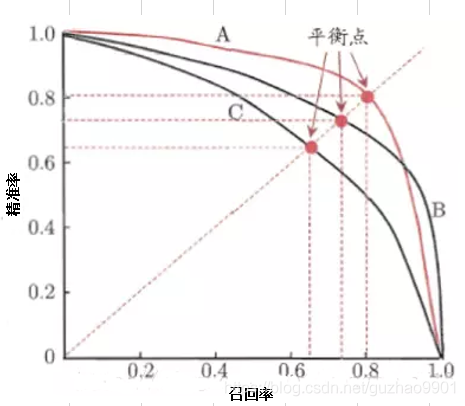如果其中一个学习器的PR曲线A完全包住另一个学习器B的PR曲线，则可断言A的性能优于B。但是A和B发生交叉，那性能该如何判断呢？我们可以根据曲线下方的面积大小来进行比较，但更常用的是平衡点F1。平衡点（BEP）是P=R时的取值（斜率为1），F1值越大，我们可以认为该学习器的性能较好。F1的计算如下所示：F1 = 2 * P * R ／( P + R )

• 非VOC2007的算法：对于某类下全部的真实目标，将IOU>=0.5 的作为检测出来的目标，取不同的confidence 阈值计算对应的precision 和recall，对于每个recall，取其对应的最大precision，对这些precision 求平均即为该类的AP 值。所有类的AP 值求平均即为mAP。
• 在评测时，COCO评估了在不同的交并比(IoU)[0.5:0.05:0.95]共10个IoU下的AP，并且在最后以这些阈值下的AP平均作为结果，记为mAP@[.5, .95]。而在Pascal VOC中，检测结果只评测了IOU在0.5这个阈值下的AP值。
因此相比VOC而言，COCO数据集的评测会更加全面：不仅评估到物体检测模型的分类能力，同时也能体现出检测模型的定位能力。因此在IoU较大如0.8时，预测框必须和真实的框具有很大的重叠比才能被视为正确。

要计算mAP必须先绘出各类别PR曲线，计算出AP。而如何采样PR曲线，VOC采用过两种不同方法。参见：The PASCAL Visual Object Classes Challenge 2012 (VOC2012) Development Kit

1. 在VOC2010以前，只需要选取当Recall >= 0, 0.1, 0.2, …, 1共11个点时的Precision最大值，然后AP就是这11个Precision的平均值
2. 在VOC2010及以后，需要针对每一个不同的Recall值（包括0和1），选取其大于等于这些Recall值时的Precision最大值，然后计算PR曲线下面积作为AP值。

mAP计算示例：假设，对于Aeroplane类别，我们网络有以下输出(BB表示BoundingBox序号，IoU>0.5时GT=1)：
BB | confidence | GT
BB1 | 0.9 | 1
BB2 | 0.9 | 1
BB1 | 0.8 | 1
BB3 | 0.7 | 0
BB4 | 0.7 | 0
BB5 | 0.7 | 1
BB6 | 0.7 | 0
BB7 | 0.7 | 0
BB8 | 0.7 | 1
BB9 | 0.7 | 1

因此，我们有 TP=5 (BB1, BB2, BB5, BB8, BB9), FP=5 (重复检测到的BB1也算FP)。除了表里检测到的5个GT以外，我们还有2个GT没被检测到，因此: FN = 2. 这时我们就可以按照Confidence的顺序给出各处的PR值，如下：
rank=1 precision=1.00 and recall=0.14（recall = TP / (TP+FN) , 到rank=1 时，预测到的TP= 1 , 针对假设，GT有7个,因此recall = 1/7 = 0.14）
rank=2 precision=1.00 and recall=0.29
rank=3 precision=0.66 and recall=0.29
rank=4 precision=0.50 and recall=0.29
rank=5 precision=0.40 and recall=0.29
rank=6 precision=0.50 and recall=0.43
rank=7 precision=0.43 and recall=0.43
rank=8 precision=0.38 and recall=0.43
rank=9 precision=0.44 and recall=0.57
rank=10 precision=0.50 and recall=0.71

对于上述PR值，如果我们采用：

1. VOC2010之前的方法，我们选取Recall >= 0, 0.1, …, 1的11处Percision的最大值：1, 1, 1, 0.5, 0.5, 0.5, 0.5, 0.5, 0, 0, 0。此时Aeroplane类别的 AP = 5.5 / 11 = 0.5
2. VOC2010及以后的方法，对于Recall >= 0, 0.14, 0.29, 0.43, 0.57, 0.71, 1，我们选取此时Percision的最大值：1, 1, 1, 0.5, 0.5, 0.5, 0。此时Aeroplane类别的 AP = (0.14-0)*1 + (0.29-0.14)*1 + (0.43-0.29)*0.5 + (0.57-0.43)*0.5 + (0.71-0.57)*0.5 + (1-0.71)*0 = 0.5
mAP就是对每一个类别都计算出AP然后再计算AP平均值就好了

参考资料
https://www.zhihu.com/question/53405779
https://blog.csdn.net/guzhao9901/article/details/107961184

展开全文• 在进行图像显著性分析时，要通过PR曲线进行比较不同方法的好坏，这个源码可以实现此功能。
• ## AUC PR曲线 学习曲线

千次阅读 2021-04-23 15:19:42
AUC（Area Under Curve）被定义为ROC曲线下的面积。我们往往使用AUC值作为模型的评价标准是因为很多时候ROC曲线并不能清晰的说明哪个分类器的效果更好，而作为一个数值，对应AUC更大的分类器效果更好。 其中，ROC...

AUC（Area Under Curve）被定义为ROC曲线下的面积。我们往往使用AUC值作为模型的评价标准是因为很多时候ROC曲线并不能清晰的说明哪个分类器的效果更好，而作为一个数值，对应AUC更大的分类器效果更好。

其中，ROC曲线全称为受试者工作特征曲线 （receiver operating characteristic curve），它是根据一系列不同的二分类方式（分界值或决定阈），以真阳性率（敏感性）为纵坐标，假阳性率（1-特异性）为横坐标绘制的曲线

AUC就是衡量学习器优劣的一种性能指标。从定义可知，AUC可通过对ROC曲线下各部分的面积求和而得。

## ROC曲线的意义

（1）主要作用

1.ROC曲线能很容易的查出任意阈值对学习器的泛化性能影响。

2.有助于选择最佳的阈值。ROC曲线越靠近左上角，模型的准确性就越高。最靠近左上角的ROC曲线上的点是分类错误最少的最好阈值，其假正例和假反例总数最少。

3.可以对不同的学习器比较性能。将各个学习器的ROC曲线绘制到同一坐标中，直观地鉴别优劣，靠近左上角的ROC曲所代表的学习器准确性最高。

（2）优点

1. 该方法简单、直观、通过图示可观察分析学习器的准确性，并可用肉眼作出判断。ROC曲线将真正例率和假正例率以图示方法结合在一起，可准确反映某种学习器真正例率和假正例率的关系，是检测准确性的综合代表。

2. ROC曲线不固定阈值，允许中间状态的存在，利于使用者结合专业知识，权衡漏诊与误诊的影响，选择一个更加的阈值作为诊断参考值。

## AUC

如果两条ROC曲线没有相交，我们可以根据哪条曲线最靠近左上角哪条曲线代表的学习器性能就最好。但是，实际任务中，情况很复杂，如果两条ROC曲线发生了交叉，则很难一般性地断言谁优谁劣。在很多实际应用中，我们往往希望把学习器性能分出个高低来。在此引入AUC面积。

在进行学习器的比较时，若一个学习器的ROC曲线被另一个学习器的曲线完全“包住”，则可断言后者的性能优于前者；若两个学习器的ROC曲线发生交叉，则难以一般性的断言两者孰优孰劣。此时如果一定要进行比较，则比较合理的判断依据是比较ROC曲线下的面积，即AUC(Area Under ROC Curve)。

## AUC面积的意义

AUC是衡量二分类模型优劣的一种评价指标，表示预测的正例排在负例前面的概率。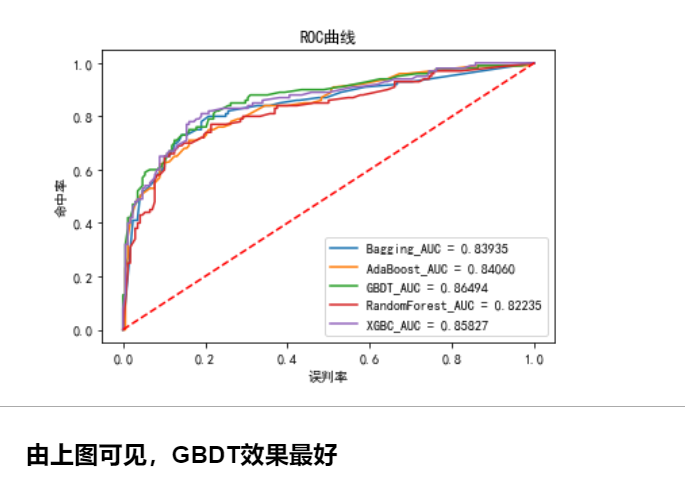# 有效性不同的模型的 ROC 曲线图示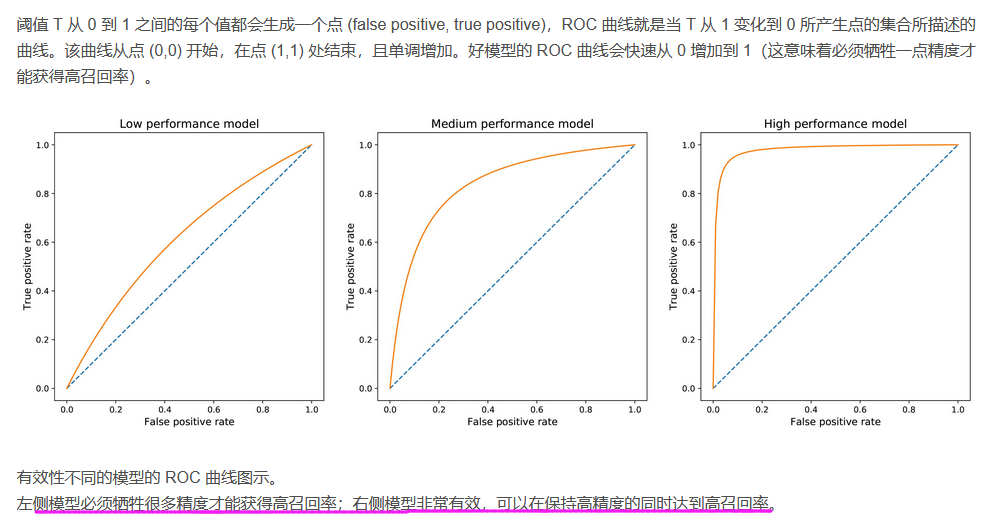from xgboost.sklearn import XGBClassifier
from sklearn.metrics import roc_curve, auc
from sklearn.model_selection import train_test_split
X_train, X_test, Y_train, Y_test = train_test_split(X, Y, test_size=0.3)
def plot_roc():
clf_bagging = BaggingClassifier()
clf_rf = RandomForestClassifier()
clf_xgb = XGBClassifier()
clfs = [clf_bagging, clf_ada, clf_gbdt, clf_rf,clf_xgb]
names = ['Bagging', 'AdaBoost', 'GBDT', 'RandomForest','XGBC']
prbs_1 = []
for clf in clfs:
clf.fit(X_train, Y_train)
pre = clf.predict(X_test)
y_prb_1 = clf.predict_proba(X_test)[:, 1]
prbs_1.append(y_prb_1)
for index, value in enumerate(prbs_1):
fpr, tpr, thresholds = roc_curve(Y_test, value)
roc_auc = auc(fpr, tpr)
plt.rcParams['font.sans-serif'] = ['SimHei']
plt.rcParams['axes.unicode_minus'] = False
plt.plot(fpr, tpr, label='{0}_AUC = {1:.5f}'.format(names[index], roc_auc))
plt.title('ROC曲线')
plt.xlim([-0.05, 1.05])
plt.ylim([-0.05, 1.05])

plt.legend(loc='lower right')
plt.plot([0, 1], [0, 1], 'r--')
plt.ylabel('命中率')
plt.xlabel('误判率')
plt.show()
plot_roc()

# K-S曲线

KS曲线是用来衡量分类型模型准确度的工具。KS曲线与ROC曲线非常的类似。其指标的计算方法与混淆矩阵、ROC基本一致。它只是用另一种方式呈现分类模型的准确性。KS值是KS图中两条线之间最大的距离，其能反映出分类器的划分能力。

一句话概括版本：

KS曲线是两条线，其横轴是阈值，纵轴是TPR与FPR。两条曲线之间之间相距最远的地方对应的阈值，就是最能划分模型的阈值。

KS值是MAX(TPR - FPR），即两曲线相距最远的距离。

KS（Kolmogorov-Smirnov）评价指标，通过衡量好坏样本累计分布之间的差值，来评估模型的风险区分能力。

KS、AUC、PR曲线对比：

1）ks和AUC一样，都是利用TPR、FPR两个指标来评价模型的整体训练效果。

2）不同之处在于，ks取的是TPR和FPR差值的最大值，能够找到一个最优的阈值；AUC只评价了模型的整体训练效果，并没有指出如何划分类别让预估的效果达到最好，就是没有找到好的切分阈值。

3）与PR曲线相比，AUC和KS受样本不均衡的影响较小，而PR受其影响较大。（详看P-R曲线及与ROC曲线区别

KS的曲线图走势大概如下：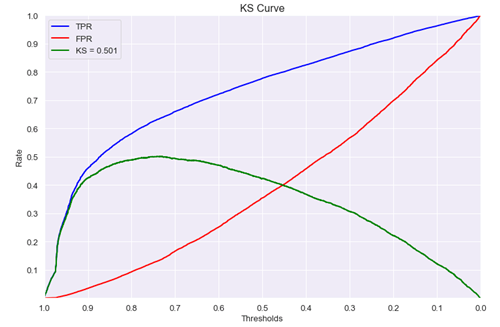ks值<0.2,一般认为模型没有区分能力。

ks值[0.2,0.3],模型具有一定区分能力，勉强可以接受

ks值[0.3,0.5],模型具有较强的区分能力。

ks值大于0.75，往往表示模型有异常。

二、直观理解KS

1）如何直观的理解ks呢？

参考上述ks曲线图，可以这样理解，随着阈值从大逐渐的变小，TPR的提升速度高于FPR的提升速度，直到一个临界阈值threshold，之后TPR提升速度低于FPR，这个临界threshold便是最佳阈值。在前期TPR越快提升，模型效果越好；反之，FPR越快提升，模型效果就越差。

# P-R曲线及与ROC曲线区别

https://www.cnblogs.com/gczr/p/10137063.html

P-R曲线刻画查准率和查全率之间的关系，查准率指的是在所有预测为正例的数据中，真正例所占的比例，查全率是指预测为真正例的数据占所有正例数据的比例。
即：查准率P=TP／(TP + FP) 查全率=TP／（TP+FN）
查准率和查全率是一对矛盾的度量，一般来说，查准率高时，查全率往往偏低，查全率高时，查准率往往偏低，例如，若希望将好瓜尽可能多选出来，则可通过增加选瓜的数量来实现，如果希望将所有的西瓜都选上，那么所有的好瓜必然都被选上了，但这样查准率就会较低；若希望选出的瓜中好瓜比例尽可能高，则可只挑选最有把握的瓜，但这样就难免会漏掉不少好瓜，使得查全率较低。

在很多情况下，我们可以根据学习器的预测结果对样例进行排序，排在前面的是学习器认为最可能是正例的样本，排在后面的是学习器认为最不可能是正例的样本，按此顺序逐个把样本作为正例进行预测，则每次可计算当前的查全率和查准率，以查准率为y轴，以查全率为x轴，可以画出下面的P-R曲线。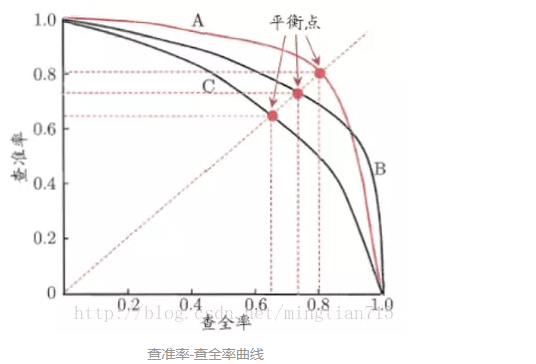如果一个学习器的P-R曲线被另一个学习器的P-R曲线完全包住，则可断言后者的性能优于前者，例如上面的A和B优于学习器C，但是A和B的性能无法直接判断，但我们往往仍希望把学习器A和学习器B进行一个比较，我们可以根据曲线下方的面积大小来进行比较，但更常用的是平衡点或者是F1值。平衡点（BEP）是查准率=查全率时的取值，如果这个值较大，则说明学习器的性能较好。而F1 = 2 * P * R ／( P + R )，同样，F1值越大，我们可以认为该学习器的性能较好。

联合建模(BJ)P-R曲线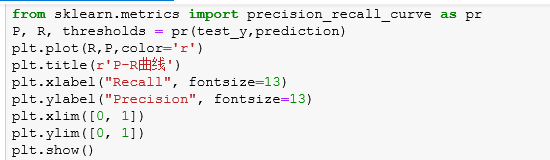参数：

y_true：array, shape = [n_samples]：真实标签。如果标签不是{-1,1}或{0,1}，那么pos_label应该显式给出。
probas_pred：array, shape = [n_samples] ：正类的预测概率或决策函数
pos_label：int or str, default=None：正类标签。当pos_label=None时，如果y_true为{- 1,1}或{0,1}，则pos_label设置为1，否则将报错。

返回值：

precision：array, shape = [n_thresholds + 1]：精度，最后一个元素是1。
recall：array, shape = [n_thresholds + 1]：召回率，最后一个是0
thresholds：array, shape = [n_thresholds <= len(np.unique(probas_pred))]：用于计算精度和召回率的决策函数的阈值

例子：

import numpy as np
from sklearn.metrics import precision_recall_curve
y_true = np.array([0, 0, 1, 1])
y_scores = np.array([0.1, 0.4, 0.35, 0.8])
precision, recall, thresholds = precision_recall_curve(y_true, y_scores)

如何选择呢？

• 在很多实际问题中，正负样本数量往往很不均衡。比如，计算广告领域经常涉及转化率模型，正样本的数量往往是负样本数量的1/1000，甚至1/10000。若选择不同的测试集，P-R曲线的变化就会非常大，而ROC曲线则能够更加稳定地反映模型本身的好坏。所以，ROC曲线的适用场景更多，被广泛用于排序、推荐、广告等领域。
• 但需要注意的是，选择P-R曲线还是ROC曲线是因实际问题而异的，如果研究者希望更多地看到模型在特定数据集上的表现，P-R曲线则能够更直观地反映其性能
• PR曲线比ROC曲线更加关注正样本，而ROC则兼顾了两者。
• AUC越大，反映出正样本的预测结果更加靠前。（推荐的样本更能符合用户的喜好）
• 正负样本比例失调时，比如正样本1个，负样本100个，则ROC曲线变化不大，此时用PR曲线更加能反映出分类器性能的好坏。这个时候指的是两个分类器，因为只有一个正样本，所以在画auc的时候变化可能不太大；但是在画PR曲线的时候，因为要召回这一个正样本，看哪个分类器同时召回了更少的负样本，差的分类器就会召回更多的负样本，这样precision必然大幅下降，这样分类器性能对比就出来了。

# 学习曲线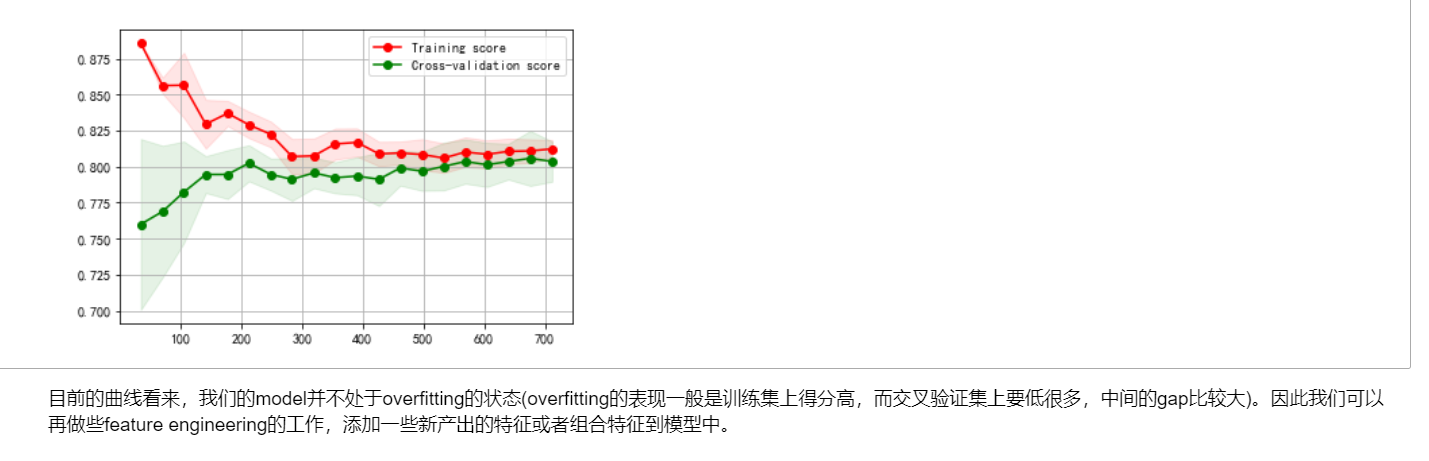import numpy as np
import matplotlib.pyplot as plt
from sklearn.model_selection import learning_curve

# 用sklearn的learning_curve得到training_score和cv_score，使用matplotlib画出learning curve
def plot_learning_curve(estimator, title, X, y, ylim=None, cv=None, n_jobs=1,
train_sizes=np.linspace(.05, 1., 20), verbose=0):
"""
画出data在某模型上的learning curve.
参数解释
----------
estimator : 你用的分类器。
title : 表格的标题。
X : 输入的feature，numpy类型
y : 输入的target vector
ylim : tuple格式的(ymin, ymax), 设定图像中纵坐标的最低点和最高点
cv : 做cross-validation的时候，数据分成的份数，其中一份作为cv集，其余n-1份作为training(默认为3份)
n_jobs : 并行的的任务数(默认1)
"""
train_sizes, train_scores, test_scores = learning_curve(
estimator, X, y, cv=cv, n_jobs=n_jobs, train_sizes=train_sizes)

train_scores_mean = np.mean(train_scores, axis=1)
train_scores_std = np.std(train_scores, axis=1)
test_scores_mean = np.mean(test_scores, axis=1)
test_scores_std = np.std(test_scores, axis=1)

plt.grid()
plt.fill_between(train_sizes, train_scores_mean - train_scores_std,
train_scores_mean + train_scores_std, alpha=0.1,
color="r")
plt.fill_between(train_sizes, test_scores_mean - test_scores_std,
test_scores_mean + test_scores_std, alpha=0.1, color="g")
plt.plot(train_sizes, train_scores_mean, 'o-', color="r",
label="Training score")
plt.plot(train_sizes, test_scores_mean, 'o-', color="g",
label="Cross-validation score")

plt.legend(loc="best")
return plt

plot_learning_curve(LR, u"学习曲线",x, y)

展开全文• ## PR曲线与ROC曲线

千次阅读 2021-03-18 20:07:49
PR曲线概念 PR曲线中的P代表的是precision（精准率），R代表的是recall（召回率），其代表的是精准率与召回率的关系，一般情况下，将recall设置为横坐标，precision设置为纵坐标。 PR曲线功能说明 一条PR曲线要对应...

## PR曲线概念

PR曲线中的P代表的是precision（精准率），R代表的是recall（召回率），其代表的是精准率与召回率的关系，一般情况下，将recall设置为横坐标，precision设置为纵坐标。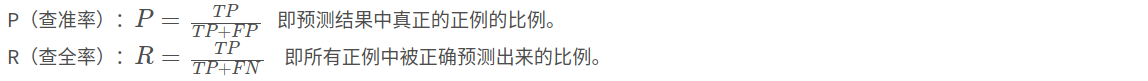## P-R曲线怎么画？

在机器学习中，分类器往往输出的不是类别标号，而是属于某个类别的概率值，根据分类器的预测结果从大到小对样例进行排序，排在前面的是学习器认为最可能是正例的样本，排在后面的是学习器认为最不可能是正例的样本，可以选取不同的阈值或者按此顺序逐个把样本作为正例进行预测，则每次可计算当前的P、R值，以P为y轴，以R为x轴，可以画出P-R曲线。

• 绘制：选取不同的confidence置信度阈值，可以在PR坐标系上得到不同的点，连接这些点即可获得PR曲线
• 用途：用来评估模型性能。Precision值和Recall值越大越好，所以PR曲线越往右上角凸越好。

举例：

Inst#是样本序号，图中有20个样本。Class是ground truth 标签，p是positive样本（正例）,n当然就是negative（负例） score是我的分类器对于该样本属于正例的可能性的打分。因为一般模型输出的不是0,1的标注，而是小数，相当于置信度。

然后设置一个从高到低的阈值T，大于等于阈值T的被我正式标注为正例，小于阈值T的被我正式标注为负例。

显然，我设置n个阈值，我就能得到n种标注结果，评判我的模型好不好使。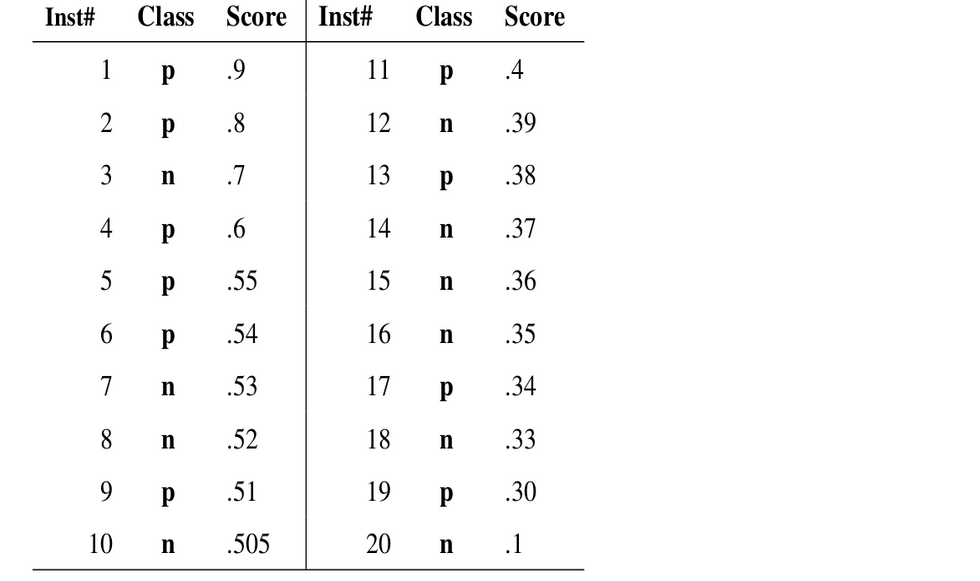如上图：真实情况 正例p 反例n 各有10个。

0.9作为阈值（大于等于0.9为正例，小于0.9为反例），此时TP=1，FP=0，FN=9，故P=1，R=0.1。
用0.8作为阈值，此时TP=2，FP=0，FN=8，P=1， R=0.2。
用0.7作为阈值，此时TP=2，FP=1，FN=7，P=0.67，R=0.2。
用0.6作为阈值，此时TP=3，FP=1，FN=6，P=0.75，R=0.3。
以此类推。。。
最后得到一系列P、R值序列，就画出P-R曲线（示意图，不对应上面数据）：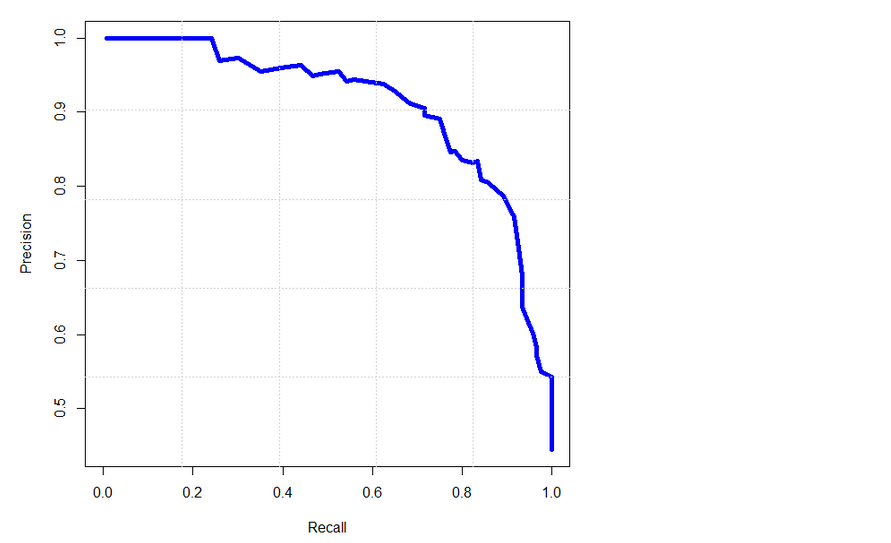## P-R曲线解析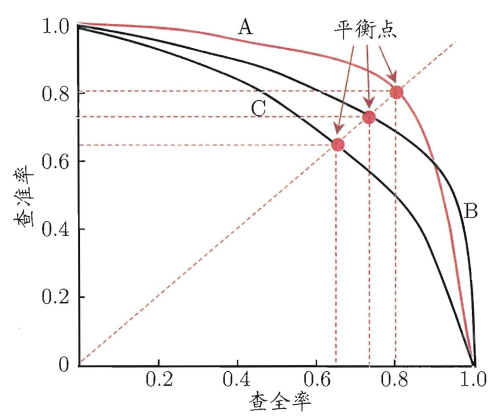显然，P-R 曲线越靠近右上角性能越好。

如果一个学习器的P-R曲线被另一个学习器的P-R曲线完全包住，则可断言后者的性能优于前者，例如上面的A和B优于学习器C。但是A和B的性能无法直接判断，我们可以根据曲线下方的面积大小来进行比较，但更常用的是平衡点或者是F1-score

平衡点（BEP）是P=R时的取值，如果这个值较大，则说明学习器的性能较好。

F1 = 2 * P * R ／( P + R )，F1-score综合考虑了P值和R值，是精准率和召回率的调和平均值， 同样，F1值越大，我们可以认为该学习器的性能较好。

## ROC曲线

在ROC曲线中，横轴是假正例率（FPR），纵轴是真正例率（TPR）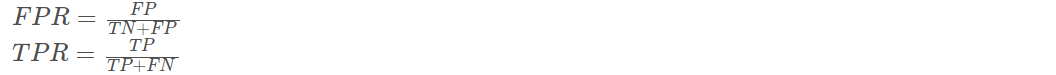(1)真正类率(True Postive Rate)，代表分类器预测的正类中实际正实例占所有正实例的比例。
(2)负正类率(False Postive Rate)，代表分类器预测的正类中实际负实例占所有负实例的比例。
我们可以发现：TPR=Recall

ROC曲线也需要相应的阈值才可以进行绘制，原理同上的PR曲线。
用0.9作为阈值，此时TP=1，FP=0，FN=9，TN=10，故TPR=0.1，FPR=0。
用0.8作为阈值，此时TP=2，FP=0，FN=8，TN=10，故TPR=0.2，FPR=0。
用0.7作为阈值，此时TP=2，FP=1，FN=8，TN=9，故TPR=0.2，FPR=0.1。
用0.6作为阈值，此时TP=3，FP=1，FN=7，TN=9，故TPR=0.3，FPR=0.1。
以此类推。。。
最后的ROC曲线如下图：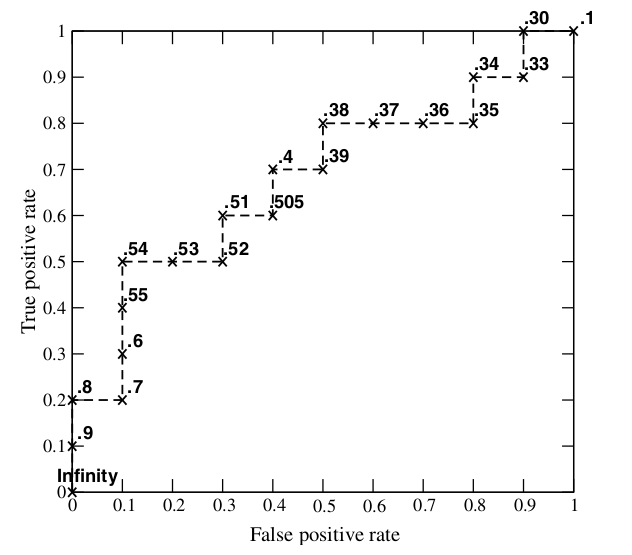ROC曲线图中的四个点
第一个点：(0,1)，即FPR=0, TPR=1，这意味着FN=0，并且FP=0。这是完美的分类器，它将所有的样本都正确分类。
第二个点：(1,0)，即FPR=1，TPR=0，类似地分析可以发现这是一个最糟糕的分类器，因为它成功避开了所有的正确答案。
第三个点：(0,0)，即FPR=TPR=0，即FP=TP=0，可以发现该分类器预测所有的样本都为负样本（negative）。
第四个点：(1,1)，分类器实际上预测所有的样本都为正样本。经过以上的分析，ROC曲线越接近左上角，该分类器的性能越好。

一个对比：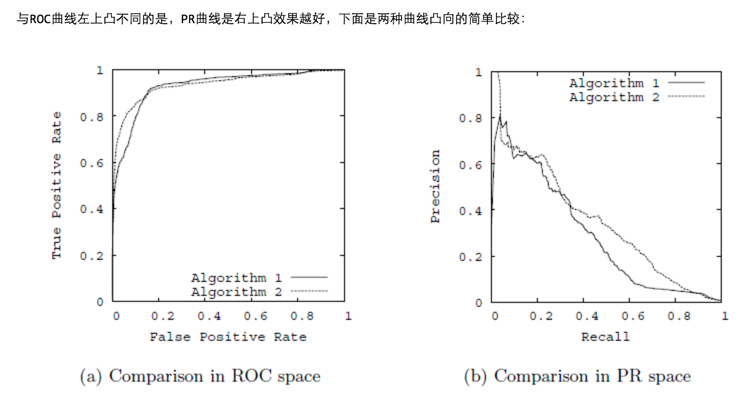## AUC

这里补充一下AUC的简单介绍。
AUC (Area under Curve)：ROC曲线下的面积，介于0.1和1之间，作为数值可以直观的评价分类器的好坏，值越大越好。
AUC = 1，是完美分类器，采用这个预测模型时，存在至少一个阈值能得出完美预测。绝大多数预测的场合，不存在完美分类器。
0.5 < AUC < 1，优于随机猜测。这个分类器（模型）妥善设定阈值的话，能有预测价值。
AUC = 0.5，跟随机猜测一样（例：丢铜板），模型没有预测价值

## PR曲线和ROC曲线的关系

PR曲线和ROC曲线都能评价分类器的性能。如果分类器a的PR曲线或ROC曲线包围了分类器b对应的曲线，那么分类器a的性能好于分类器b的性能。
PR曲线和ROC曲线有什么联系和不同：
相同点：
首先从定义上PR曲线的R值是等于ROC曲线中的TPR值。
都是用来评价分类器的性能的。
不同点：
ROC曲线是单调的而PR曲线不是（根据它能更方便调参），可以用AUC的值得大小来评价分类器的好坏（是否可以用PR曲线围成面积大小来评价呢？）。
正负样本的分布失衡的时候，ROC曲线保持不变，而PR曲线会产生很大的变化。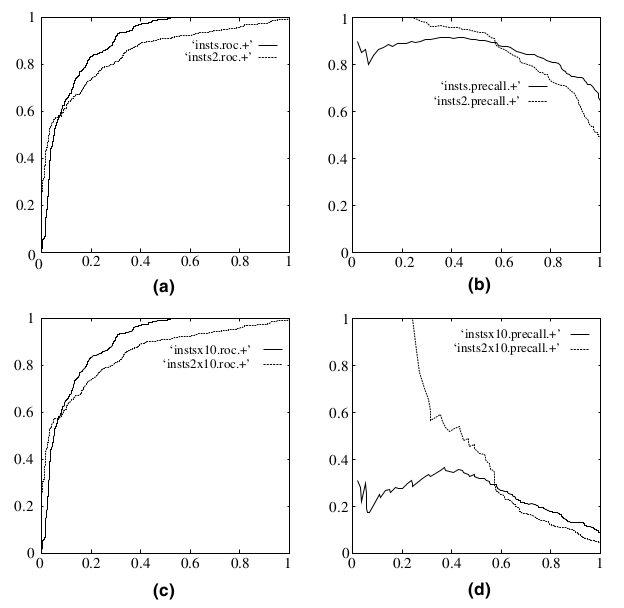（a）（b）分别是正反例相等的时候的ROC曲线和PR曲线
（c）（d）分别是十倍反例一倍正例的ROC曲线和PR曲线
可以看出，在正负失衡的情况下，从ROC曲线看分类器的表现仍然较好（图c），然而从PR曲线来看，分类器就表现的很差。
事实情况是分类器确实表现的不好（分析过程见知乎 qian lv 的回答），是ROC曲线欺骗了我们。

参考（感谢）
https://blog.csdn.net/teminusign/article/details/51982877
https://www.jianshu.com/p/ac46cb7e6f87
http://www.fullstackdevel.com/computer-tec/data-mining-machine-learning/501.html

展开全文• 分类、检索中的评价指标很多，Precision、Recall、Accuracy、F1、ROC、PR Curve......一、历史wiki上说，ROC曲线最先在二战中分析雷达信号，用来检测敌军。诱因是珍珠港事件；由于比较有用，慢慢用到了心理学、医学...
• 1.ROC曲线 TPR=TPP=TPTP+FNTPR = \frac{TP}{P} = \frac{TP}{TP + FN}TPR=PTP​=TP+FNTP​ FPR=FPN=FPFP+TNFPR = \frac{FP}{N} = \frac{FP}{FP + TN}FPR=NFP​=FP+TNFP​ ROC曲线的纵坐标为TPR，真正率，其实也是召回...ROC曲线 PR曲线
• 研究了三天的多分类pr曲线问题终于在昨天晚上凌晨一点绘制成功了！！ 现将所学所感记录一下，一来怕自己会忘可以温故一下，二来希望能给同样有疑惑的铁子们一些启迪！ 下图为我画的pr曲线，因为准确度超过了97%，...python 分类
• 本篇博客主要是介绍PR曲线与目标检测中的mAP指标。目标检测 python 人工智能
• 介绍ROC和PR前先介绍混淆矩阵便于理解。 - 预测值 正 预测值 负 真实值 正 TP FN 真实值 负 FP TN TP(True Positive): 被判定为正样本，实际也为正样本 FN(False Negative):伪阴性 ，被判定为负样本，...机器学习 人工智能
• 用的yolov5 5.0版本 训练完以后会有一个pr曲线图，一共5个类，但是自动生成的这个曲线中有几类目标的曲线不想要 请问怎么生成只想要的几个类的pr曲线python 目标检测
• # @brief : 如何绘制PR曲线 # @author : liupc # @date : 2021/8/2 import numpy as np from tqdm import tqdm import matplotlib.pyplot as plt #计算汉明距离。有几位不同，距离就为几。 def CalcHammingDist...PR曲线
• 是不是对一堆PR曲线、ROC曲线、AUC、AP傻傻分不清楚？是不是学完就忘忘了又学？希望这篇文章能帮你梳理清楚一坨乱麻。 1、混淆矩阵 每次在重温理解AUC、PR曲线、ROC曲线的时候，第一步就是在纸上画出来这个混淆矩阵...机器学习 人工智能
• _python__代码_相关文件_下载 这个 repo 是为评估二值图像分割结果而开发的。 已实施的措施 MAE 平均绝对误差 ...精确召回曲线 精确召回曲线 F-测量曲线 更多详情、使用方法，请下载后阅读README.md文件python
• 基于此，可以绘制PR曲线，python绘制PR曲线比较方便。 首先，可以使用precision_recall_curve函数得到 #选择测试数据 import pandas as pd df = pd.read_csv('file_name.csv') X = df[df.columns[:-1]] y = df[df....python 曲线 阈值
• 用的YOLOv5-5.0版本，训练完以后有一个自动生成的pr曲线，但是太粗糙了，不能用在论文里。 有没有什么方法可以画一个比较好看的pr曲线图，最好是可以将横纵坐标数据导出来，自己用其他的软件画python 目标检测 pytorch
• Python计算PR曲线绘制：二分类PR曲线绘制、多分类PR曲线绘制、平均PR值计算 目录 Python计算PR曲线绘制：二分类PR曲线绘制、多分类PR曲线绘制、平均PR值计算 #二分类的平均AP(Average precision)值计算 #二...python 数据挖掘 机器学习 自然语言处理
• ## 绘制PR曲线

千次阅读 2020-07-29 22:48:52
open(cls + '_pr.pkl','rb') inf = cPickle.load(fr) fr.close() x=inf['rec'] y=inf['prec'] fig = plt.figure(1, dpi=160) ax = fig.add_subplot(1,1,1) ax.plot(x, y, label='PR') #ax.plot(result['Avg Recall']...
• ## YOLOv4 绘制PR曲线

千次阅读 2021-03-23 21:32:02
YOLOv4 绘制PR曲线简述YOLOv4 自带mAP检测代码第三方库 简述 最近做的一项工作就是交通目标的识别，我用的是YOLOv4基于Darknet的那一版，其中最重要的评价一项目标检测，但是我试过YOLOv4自带的map和网上常用的绘制...图像识别 神经网络 机器学习
• PR方程编写，密度根求解；逸度系数求解，混合规则改进
• 闲来无事，边理解PR曲线和ROC曲线，边写了一下计算两个指标的代码。在 python 环境下，sklearn里有现成的函数计算ROC曲线坐标点，这里为了深入理解这两个指标，写代码的时候只用到numpy包。事实证明，实践是检验真理...
• ## 终于搞懂了PR曲线

千次阅读 2021-08-29 20:46:25
图中的PR曲线很奇怪, 左边从1突然变到0. PR源码分析 为了搞清楚这个问题, 对源码进行了分析. 如下所示为上图对应的代码: from sklearn.metrics import precision_recall_curve import matplotlib.pyplot as plt ...sklearn 机器学习
• ## PR曲线详解

万次阅读 多人点赞 2020-08-13 08:54:38
目录PR曲线概念precision（精准率）和recall（召回率）PR曲线功能说明 PR曲线概念 PR曲线中的P代表的是precision（精准率），R代表的是recall（召回率），其代表的是精准率与召回率的关系，一般情况下，将recall设置...机器学习 人工智能...